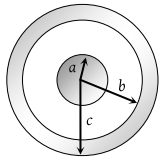You’ve reached the end of your free Videos limit.
#25 | Charged Infinite Sheet (Gauss Theorem)
(Physics) > Electric Charges and Fields
Unable to watch the video, please try another server
Related Practice Questions :

A solid conducting sphere of radius a has a net positive charge 2Q. A conducting spherical shell of inner radius b and outer radius c is concentric with the solid sphere and has a net charge –Q. The surface charge density on the inner and outer surfaces of the spherical shell will be(1) $-\frac{2Q}{4\pi {b}^{2}},\frac{Q}{4\pi {c}^{2}}$

(2) $-\frac{Q}{4\pi {b}^{2}},\frac{Q}{4\pi {c}^{2}}$

(3) $0,\frac{Q}{4\pi {c}^{2}}$

(4) None of the above

High Yielding Test Series + Question Bank - NEET 2020

Difficulty Level:

A cylinder of radius R and length L is placed in a uniform electric field E parallel to the cylinder axis. The total flux for the surface of the cylinder is given by

(1) $2\pi {R}^{2}E$

(2) $\pi {R}^{2}/E$

(3) $\left(\pi {R}^{2}-\pi R\right)/E$

(4) Zero

High Yielding Test Series + Question Bank - NEET 2020

Difficulty Level:

An electric charge is placed at the centre of a cube of side α. The electric flux on one of its faces will be

(1) $\frac{q}{6{\epsilon }_{0}}$

(2) $\frac{q}{{\epsilon }_{0}{a}^{2}}$

(3) $\frac{q}{4\pi {\epsilon }_{0}{a}^{2}}$

(4) $\frac{q}{{\epsilon }_{0}}$

High Yielding Test Series + Question Bank - NEET 2020

Difficulty Level:

Total electric flux coming out of a unit positive charge put in air is

(1) ${\epsilon }_{0}$

(2) ${\epsilon }_{0}^{-1}$

(3) ${\left(4p{\epsilon }_{0}\right)}^{-1}$

(4) $4\pi {\epsilon }_{0}$

High Yielding Test Series + Question Bank - NEET 2020

Difficulty Level:

A cube of side l is placed in a uniform field E, where $E=E\stackrel{^}{i}$. The net electric flux through the cube is

(1) Zero

(2) l2E

(3) 4l2E

(4) 6l2E